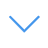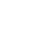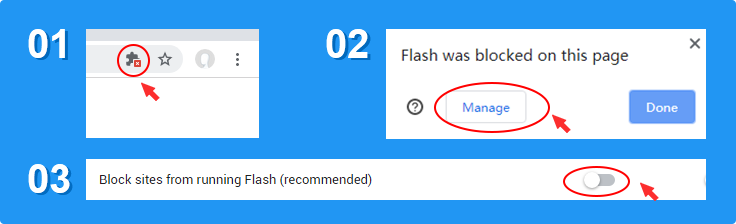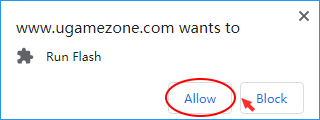# Animals Math Puzzle

Animals Math Puzzles is one of the Math Games that you can play on UGameZone.com for free. Animals Math Puzzles is mathematical game with goal to solve 50 different mathematical problems all in limited time per task. Tasks are created as linear equations with 2 and 3 variables.

Read MorePress 【Esc】 to Exit Fullscreen

# Animals Math Puzzle

Animals Math Puzzles is one of the Math Games that you can play on UGameZone.com for free. Animals Math Puzzles is mathematical game with goal to solve 50 different mathematical problems all in limited time per task. Tasks are created as linear equations with 2 and 3 variables.To play the game, you need to allow Adobe Flash Player to run in your browser.

You can use UGameZone Flash Enabler plugin to enable Flash automatically. Or you can follow our instructions to enable Flash manually.

Play Automatically

## Related Games

Step 1
Step 2